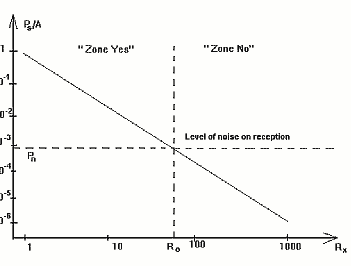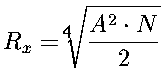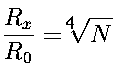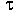## A SETI correspondent helps us to discover their signals, lost in the noise of our receivers

### N.T.Petrovich

#### Moscow Technical University of Telecommunications and Informatics, Moscow, Russia

##### Astrophysics and Space Science, 1997, vol 252, p. 55-66

It could be that SETI signals have arrived at the Earth, but are lower then the noise level, and our receivers cannot detect them. We analyze the situation when a SETI correspondent sends signals that can be accumulated upon reception, permitting detection of even very weak signals. It is shown that using this method, we can already transmit SETI signals over our entire Galaxy.
SETI -- Signals -- Accumulation

We have no grounds to exclude the possiblity that SETI signals have safely arrived at the Earth, in spite of its location at the very brink of the Galaxy. However, the levels of these signals may be lower them the total noise level at the input of our receivers. This may be one reason why the intelligent Cosmos is maintaining its silence.

Let some civilization X radiate SETI signals of power Ps. If they are sent from a star at a distance Rx, the power of these signals will be (Fig. 1)Fig. 1

 Ps = A / Rx2 1

where A is a constant depending on the parameters of the transmitter. Figure 1 also shows the input noise level Pn at the receiver civilization Y that is trying to detect these signals.

Let us denote the critical distance when Ps=Pn, Rx=R0. In particular, let us assume that reception of SETI signals is possible when Rx0, and impossible when Rx>R0. It follows that two zones form around the star with civilization X: "Zone Yes", where its SETI signal can be detected, and "Zone No", where its signals are hidden by noise (Fig. 1). The border of these zones R0 is a function of the transmitter power Ps, the transmitting and receiving antennas, the input noise level of the receiver Pn, etc. Let us suppose that civilizations X and Y use every possible means to increase R0, but without result: receiver civilization Y is still found in "Zone No". Is there any possibility to receive the SETI signals in "Zone No"?

There is only one SOLE POSSIBILITY for signal reception with signal/noise ratio arbitrarily low. It is necessary to take many measurements \$N\$ of the mixture of signal and noise, in which the correlation between signals will be higher then the correlation between the noise, and to sum them afterwards. This is a well-known method of accumulating data.Fig. 2

It is logical to suppose that other civilizations understand this SOLE POSSIBILITY no worse then we do, and very likely already send SETI signals that can be accumulated (Petrovich,(1995a)(1995b)). The simplest of these are signals with long duration (analog or digital). In practice, it is more efficient to use a time factor, since the problem of SETI contact does not limit the duration of the signal element and semantic text. I believe the accumulated SETI signals will be simple. A signal element transmitting "1" may be a sinusoidal oscillation of long or very long duration (analog version), or a long packet of periodic pulses (digital version). A signal element transmitting "0" may be a pause without any signal radiation for the same duration (Fig. 2). Since coherent accumulation with SETI signals is extremely complicated, we will analyze only non-coherent accumulation by a power receiver (radiometer), shown in Fig. 3.Fig. 3

For the case of a signal deeply immersed in noise (Ps << Pn), the time T for discovery of the signal is approximately 

 T = F*d2(Pc / Pon)-2 2

where F is the band filter transmission at the output of a quadratic detector, d2 = ( Ps / Pn)out is the signal/noise ratio at the output of the accumulator, and Pon = Ps/ F is the power noise spectrum. Formula (2) does not suppose any correlation between the noise in N counts. The time T for discovery of the signal by accumulation is equal to

 T = N/2F 3

From (2) and (3), we have

 N = 2d2(Pn / Ps)in2 4

where (Pn / Ps)in is the noise/signal ratio in the receiver input.

Using (1) and (4) and taking d=1, we find that5

It follows that the rise of Rx compared to R0 is6

Suppose that the SETI signal is a sinusoidal oscillation of duration T (Fig. 2b). The noise decorrelation timen at the output of a quadratic detector is (Fig. 3)n = 1/F 7

thus, the duration of one signal element "1" or "0", is

 T = N8

If the SETI signal is a long packet of periodic pulses (Fig. 2c), the duration of one signal element is

 T =2 N8

Using (6), (8), and (9), we obtain the values in Table 1, which gives the duration of a single elementary signal transmitting a "1" or "0". It follows from Table 1 that by making the signal element T longer and longer (analog or digital), it is possible to increase the radius of "Zone Yes" Rx by a factor of 10, 100, 1000 ... .Fig. 4

In Fig. 4, we show civilizations on stars y1 y2 y3 y4 the necessary magnitudes of \$N\$ to receive their SETI signals, and the required ratio Rx / R0 . The transmission of a semantic text of 100 elements, for example, requires a time equal to 100. Suppose civilization X is on the very brink of our Galaxy and has a small R0=100 light years. To cover the entire Galaxy, it is necessary to increase R0 by a factor of approximately 1000. Using, for example, a periodic pulse train with=10-9 sec (one nano sec) and duration of element signal "1" or "0" equal to T=2000 sec (0.55 hours), this increase can be realized. In this case, about 55 hours are required for a semantic SETI signal of 100 elements. If transmitting SETI signals, according to Table 1, the first discovery of these signals on reception will be made easier if elementary signals of longer duration are sent from time to time: 10T, 100T, 1000T ... .

Is it possible to realize accumulation of such signals upon reception without making them as long as shown in Table 1? In principle, it is possible. The first way is to receive the signals using many antennas and to sum them. The number of necessary antennas will be also N (if the summation is incoherent). The second way is to transmit the SETI signals at the same time on many frequencies and receive them by many antennas and receivers tuned to these frequencies. The number of necessary frequencies is also equal to N (if the summation is incoherent).

### Conclusions

1. The lack of recognition on the Earth of intelligent signals from the Cosmos may be due to the weakness of SETI signals compared to the noise level at the input of our receivers. It could be that SETI signals have arrived at our planet, but that we are in "Zone No" (Ps << Pn).
2. by transmitting longer and longer elementary signals (analog or digital), corresponding to "1" or "0", and accumulating them upon reception, it is possible to realize communication over the entire Galaxy without using super-power transmitters.
3. It is logical to suppose that some civilizations already send long SETI signals, expecting they will be accumulated at the receivers.
4. It we equip our SETI receivers with accumulating devices, it could allow us to pass from "Zone No" to "Zone Yes".

### Reference

Petrovich N.T. Radiowaves - it may be a bridge to another civilization M. Electrocommunication 1995,N2
Petrovich N.T. The problem of radio-contact with different civilization (problem SETI) M. Foreign Electronics 1995b,N2/3